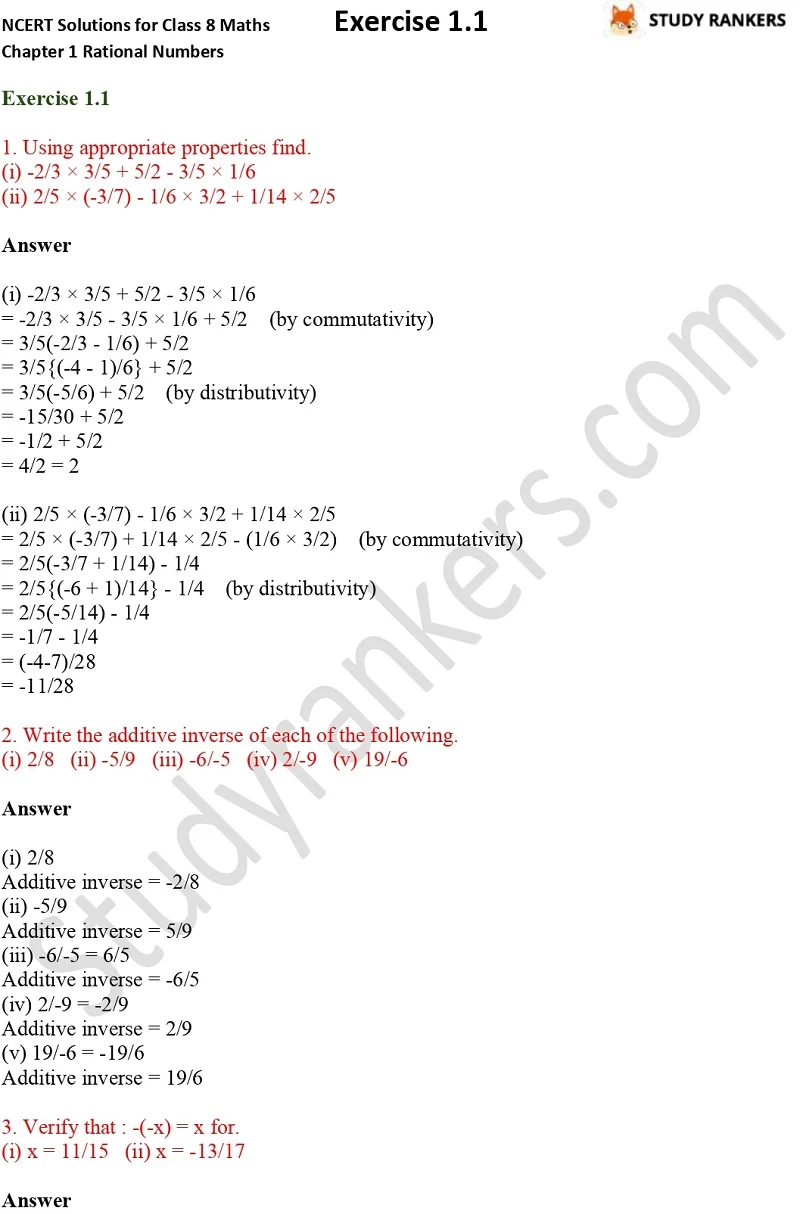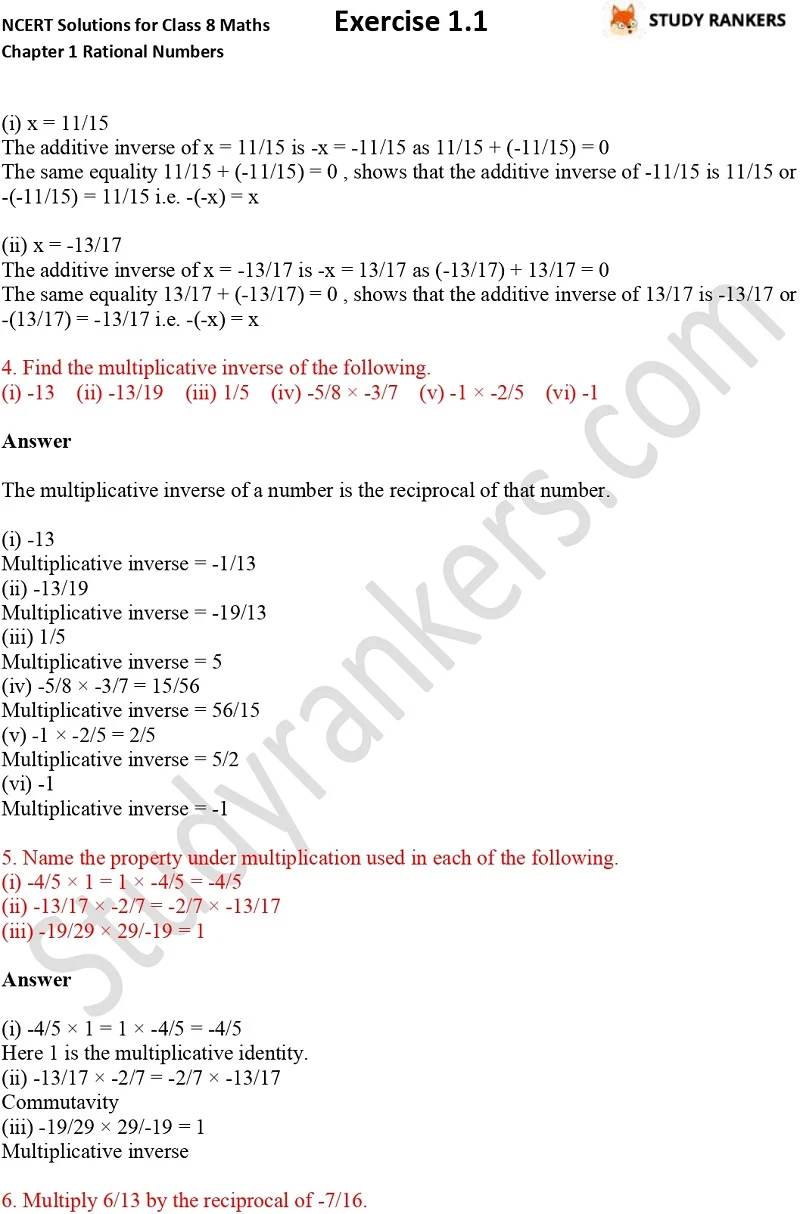## NCERT Solutions for Class 8 Maths Chapter 1 Rational Numbers Exercise 1.1

If you want NCERT Solutions for Chapter 1 Rational Numbers Exercise 1.1 Class 8 Maths then you can find them here. It will help you in knowing the basics of the chapter and completing your homework. NCERT Solutions for Class 8 Maths are very much essential for understanding what is given in the chapter and how to apply a formula correctly to solve questions. Studyrankers has prepared detailed answers of every questions so you can easily check your own.

Exercise 1.1 has total 11 questions in which you have to find multiplicative inverse etc.